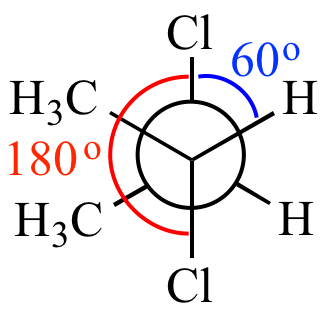Illustrated Glossary of Organic Chemistry

Dihedral angle: The angle between two planes, where each plane is defined by three atoms. In most common use, the angle between two bonds.In this Newman projection the dihedral angle between the Cl-C and H-C bonds is 60o (they are gauche), whereas the dihedral angle between the two C-Cl bonds is 180o (they are anti-periplanar).